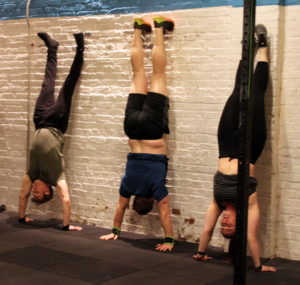## Tuesday, September 10, 2019

### Tuesday, September 10, 2019as many repetitions as possible in eight minutes:
30x double under
10x wall ball @ 20/14-lbs., 10-ft. target

### 1 Comment

1.–Scores–
Aaron B.: 5 rounds +1 wall ball (sub: 40″ bike)
Al C.: 8 rounds + 10 du
Charles B.: 6 rounds + 9 wall ball (sub: 40″ du practice)
Chase H.: 7 rounds + 25 du
Andrew Z.: 8 rounds + 16 du
Borja G.: 7 rounds + 30 du
Walt C.: 6 rounds
Anu B.: 7 rounds + 2 du
Peter Mc.: 9 rounds
David C.: 7 rounds (sub: bike 40″, med ball thruster)
Jon K.: 4 rounds (sub: 40″ du practice, 78, 8′ target)
Morgan W.: 6 rounds (sub: 40″ du practice, 72)
Bhavesh S.: 8 rounds
Dave R.: 9 rounds
Jason O.: 9 rounds + 7 du
Henry T.: 5 rounds (sub: 40″ du practice, 14-lbs.)
Kate G.: 5 rounds + 8 WB (sub: 40″ DU practice, 10-lbs med ball)
Cristian D.: 5 rounds + 7 du (sub: 40″ du practice, 106 du, 14-lbs. wall ball push press)
Buffy M.: 5 rounds
JP S.: 5 round + 8 wall ball (sub: 40″ du practice, 130 du, 14-lbs. wall ball)
Matthew M.: 7 rounds
Gabriel F.: 6 rounds + 7 du (sub: 40″ du practice, 114du)
Brian F.: 5 + 22 du (14-lbs. wall ball)
Joe T.: 7 rounds + 30 du
Melody F.: 8 rounds + 8 du
Chris H.: 9 rounds
Joya TD: 6 rounds + 2 wall ball
Anna Go.: 6 rounds (sub: 40′ du practice 70 du)
Dan D.: 6 rounds + 1 du (sub: 40″ du practice 155 du)
Charlie S.: 8 rounds + 22 du
Andrew B.: 6 rounds + 30 du (sub: 14 lb wall ball)
John S.: 5 rounds + 14 du
Alli P.: 8 rounds + 10 du
Tommy W.: 6 rounds (sub: 40″ du practice, 58 du)
Will H.: 5 rounds + 30 sec bike (sub: 40″ bike, 20-lbs. db shoulder press)
Franciso L.: 7 rounds + 30 du
Bill G.: 7 rounds + 6 du
Alex S.: 6 rounds (sub: 14-lbs. wall ball, 40″ du practice, 52 du)
Josh M.: 6 rounds
Sid: 6 rounds
RJ A.: 6 rounds (sub: 14-lbs. wall ball, 40″ du practice, 42)
Mike C.: 3 rounds + 13 du (sub: 14-lbs. wall ball)
Sergio L.: 6 rounds (sub: 14-lbs. wall ball, 40″ du practice, 13)
Dan C.: 5 rounds + 13 du
Tarek K.: 6 rounds (sub: 14-lbs. wall ball, 40″ du practice, 18)
Alex M.: 8 rounds + 7 wall ball
Adriana B.: 6 rounds + 30 du
Beth K.: 6 rounds (sub: 10-lbs. med ball thruster)
Chris C.: 8 rounds
Tara S.: 7 rounds + 30 du
Andy G.: 6 rounds + 7 wall ball (sub: 40″ du practice, 25)
Terry A.: 3 rounds + 4 wall ball (sub: 10-lbs. wall ball, 40″ du practice, 30 )
David B.: 6 rounds + 1 du (sub: 14-lbs. wall ball, 40″ du practice, 57)
Matt K.: 6 rounds + 5 du (sub: 40″ du practice, 60)
Lily S.: 5 rounds + 3 du (sub: 10-lbs. wall ball, 40″ du practice 55)
Patrick J.: 6 rounds + 6 du
Amy S.: 8 rounds + 15 du
Seth B.: 6 rounds (sub: 40″ du practice, 89)